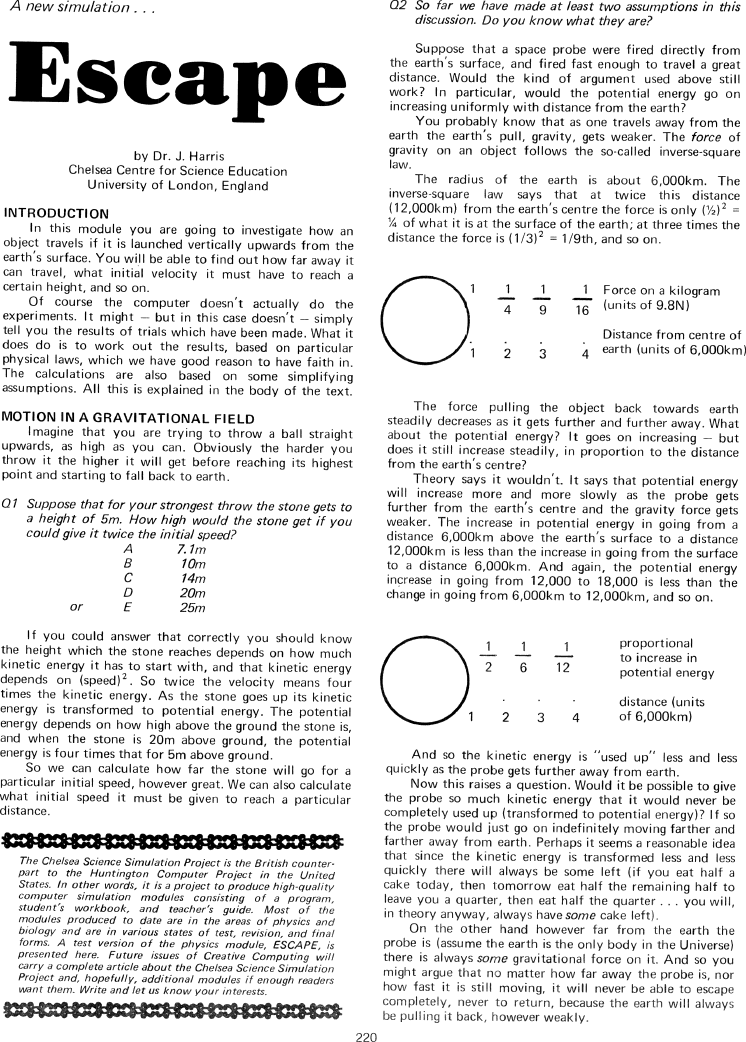The Best of Creative Computing Volume 1 (published 1976)

 `Page 220 << PREVIOUS >> NEXT Jump to page: Go to contents Go to thumbnails`

Escape (how an object travels if it is launched vertically upwards from the earth's surface, Chelsea Science Simulation Project)```A new simulation . .

Escape

by Dr. J. Harris
Chelsea Centre for Science Education
University of London, England

INTRODUCTION
ln this module you are going to investigate how an
object travels if it is launched vertically upwards from the
earth's surface. You will be able to find out how far away it
can travel, what initial velocity it must have to reach a
certain height, and so on.

Of course the computer doesn't actually do the
experiments. It might - but in this case doesn't - simply
tell you the results of trials which have been made. What it
does do is to work out the results, based on particular
physical laws, which we have good reason to have faith in.

The calculations are also based on some simplifying
assumptions. All this is explained in the body of the text.

MOTION IN A GRAVITATIONAL FIELD
Imagine that you are trying to throw a ball straight
upwards, as high as you can. Obviously the harder you
throw it the higher it will get before reaching its highest
point and starting to fall back to earth.

Q1 Suppose that for your strongest throw the stone gets to
a height of 5m. How high would the stone get if you
could give it twice the initial speed?

A       7.1m
B       10m
C       14m
D       20m
or  E       25m

If you could answer that correctly you should know
the height which the stone reaches depends on how much
depends on (speed)^2. So twice the velocity means four
times the kinetic energy. As the stone goes up its kinetic
energy is transformed to potential energy. The potential
energy depends on how high above the ground the stone is,
and when the stone is 20m above ground, the potential
energy is four times that for 5m above ground.

So we can calculate how far the stone will go for a
particular initial speed, however great. We can also calculate
what initial speed it must be given to reach a particular
distance.

*****************************************************************
The Chelsea Science Simulation Project is the British counterpart
to the Huntington Computer Project in the United
States. In other words, it is a project to produce high-quality
computer simulation modules consisting of a program,
student's workbook, and teacher's guide. Most of the
modules produced to date are in the areas of physics and
biology and are in various states of test, revision, and final
forms. A test version of the physics module, ESCAPE, is
presented here. Future issues of Creative Computing will
carry a complete article about the Chelsea Science Simulation
Mant them. Write and let us know your interests.

*****************************************************************

Q2 So far we have made at least two assumptions in this
discussion. Do you know what they are?

Suppose that a space probe were fired directly from
the earth's surface, and fired fast enough to travel a great
distance. Would the kind of argument used above still
work? In particular, would the potential energy go on
increasing uniformly with distance from the earth?

You probably know that as one travels away from the
earth the earth's pull, gravity, gets weaker. The force of
gravity on an object follows the so-called inverse-square
law.

inverse-square law says that at twice this distance
(l2,000km) from the earth's centre the force is only
(1/2)^2 = 1/4 of what it is at the surface of the earth;
at three times the distance the force is (1/3)2 = 1/9th, and so on.

1   1/4   1/9   1/16 Force on a kilogram (units of 9.8N)

1    2     3     4   Distance from centre of earth (units of  6,000km)

The force pulling the object back towards earth
steadily decreases as it gets further and further away. What
about the potential energy? It goes on increasing - but
does it still increase steadily, in proportion to the distance
from the earth's centre?

Theory says it wouldn't. It says that potential energy
will increase more and more slowly as the probe gets
further from the earth's centre and the gravity force gets
weaker. The increase in potential energy in going from a
distance 6,000km above the earth's surface to a distance
12,000km is less than the increase in going from the surface
to a distance 6,000km. And again, the potential energy
increase in going from 12,000 to 18,000 is less than the
change in going from 6,000km to 12,000km, and so on.

1/2   1/6   1/12    proportional to increase in potential energy

1    2     3     4   distance (units of  6,000km)

And so the kinetic energy is "used up" less and less
quickly as the probe gets further away from earth.

Now this raises a question. Would it be possible to give
the probe so much kinetic energy that it would never be
completely used up (transformed to potential energy)? lf so
the probe would just go on indefinitely moving farther and
farther away from earth. Perhaps it seems a reasonable idea
that since the kinetic energy is transformed less and less
quickly there will always be some left (if you eat half a
cake today, then tomorrow eat half the remaining half to
leave you a quarter, then eat half the quarter... you will,
in theory anyway, always have some cake left).

On the other hand however far from the earth the
probe is (assume the earth is the only body in the Universe)
there is always some gravitational force on it. And so you
might argue that no matter how far away the probe is, nor
how fast it is still moving, it will never be able to escape
completely, never to return, because the earth will always
be pulling it back, however weakly.Page 220        << PREVIOUS        >> NEXT        Jump to page:                Go to contents        Go to thumbnails

<!--